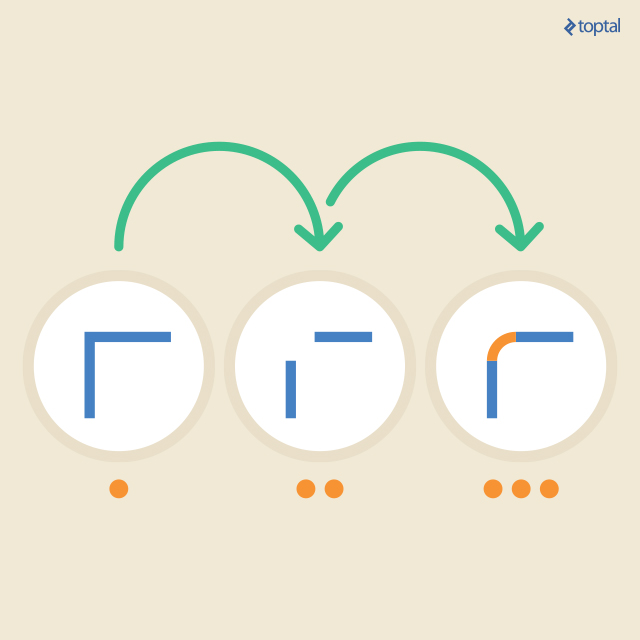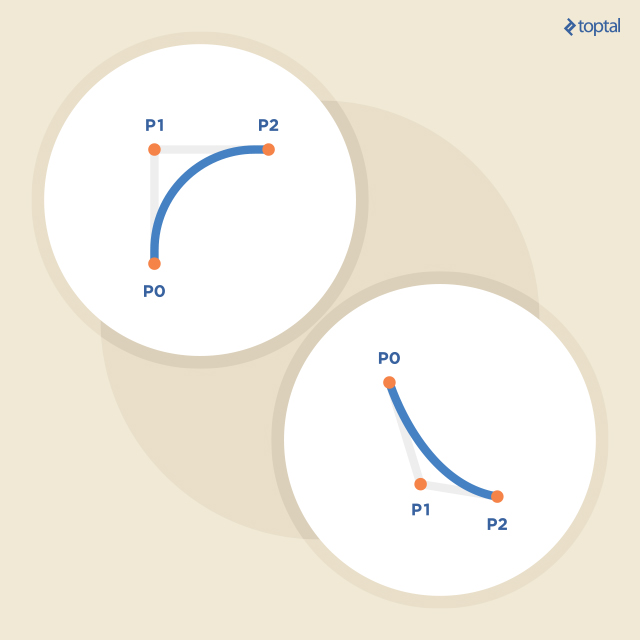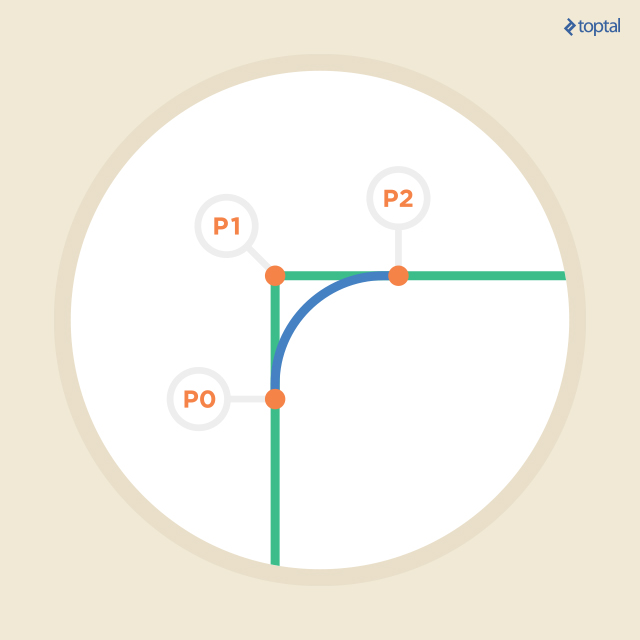Back-end

# How to Get Rounded Corner Shapes In C++ Using Bezier Curves and QPainter: A Step by Step Guide

A PhD (CE) and former assistant prof with more than 20 years of experience, Bojan's a true C/C++, Linux, and Qt expert.

## Introduction

The current trend in graphics design is to use lots of rounded corners in all sorts of shapes. We can observe this fact on many web pages, mobile devices, and desktop applications. The most notable examples are the application push buttons, which are used to trigger some action when clicked. Instead of strictly rectangular shape with 90-degree angles in the corners, they are often drawn with rounded corners. Rounded corners make the user interface feel smoother and nicer. I am not entirely convinced about this, but my designer friend tells me so.Rounded corners make the user interface feel smoother and nicer.

The visual elements of user interfaces are created by designers, and the programmer only has to put them in the right places. But what happens, when we have to generate a shape with rounded corners on the fly, and we cannot preload it? Some programming libraries offer limited capabilities for creating predefined shapes with rounded corners, but usually, they cannot be used in more complicated cases. For example, Qt framework has a class `QPainter`, which is used to draw on all classes derived from `QPaintDevice`, including widgets, pixmaps, and images. It has a method called `drawRoundedRect`, which, just as the name suggests, draws a rectangle with rounded corners. But if we need a little more complex shape, we have to implement it ourselves. How could we do that with a polygon, a planar shape bounded by a group of straight line segments? If we have a polygon drawn with a pencil on a piece of paper, my first idea would be to use an eraser and delete a small part of the lines at each corner and then connect the remaining segment ends with a circular arc. The entire process can be illustrated in the figure below.Class `QPainter` has some overloaded methods named `drawArc`, which can draw circular arcs. All of them require parameters, which define the arc center and size, starting angle and the arc length. While it is easy to determine the necessary values of these parameters for a non-rotated rectangle, it is an entirely different matter when we are dealing with more complex polygons. Plus, we would have to repeat this calculation for every polygon vertex. This calculation is a lengthy and tiresome task, and humans are prone to all sorts of calculation errors in the process. However, it is the software developers’ job to make computers work for human beings, and not vice-versa. So, here I am going to show how to develop a simple class, which can turn a complex polygon into a shape with rounded corners. Users of this class will only have to append polygon vertices, and the class will do the rest. The essential mathematical tool I use for this task, is the Bezier curve.

## Bezier curves

There are lots of mathematical books and internet resources describing the theory of Bezier curves, so I will briefly outline the relevant properties.

By definition, the Bezier curve is a curve between two points on a two-dimensional surface, the trajectory of which is governed by one or more control points. Strictly speaking, a curve between two points with no additional control points, is also a Bezier curve. However, as this results in a straight line between the two points, it is not particularly interesting, nor useful.

Quadratic Bezier curves have one control point. The theory says that a quadratic Bezier curve between points P0 and P2 with control point P1 is defined as follows:

B(t) = (1 - t)2P0 + 2t(1 - t)P1 + t2P2, where 0 ≤ t ≤ 1 (1)

So when t is equal to 0, B(t) will yield P0, when t is equal to 1, B(t) will yield P2, but in every other case, the value of B(t) will also depend on P1. Since the expression 2t(1 - t) has a maximal value at t = 0.5, that’s where the influence of P1 on B(t) will be the greatest. We can think of P1 as of an imaginary source of gravity, which pulls the function trajectory towards itself. The figure below shows a few examples of quadratic Bezier curves with their start, end and control points.So, how do we solve our problem using Bezier curves? The figure below offers an explanation.If we imagine deleting a polygon vertex and a short part of connected line segments in its surroundings, we can think of one line segment end as of P0, the other line segment end as of P2 and the deleted vertex as of P1. We apply a quadratic Bezier curve to this set of points and voila, there is the desired rounded corner.

## C++/Qt implementation using QPainter

Class `QPainter` does not have a way to draw quadratic Bezier curves. While it is quite easy to implement it from scratch following the equation (1), the Qt library does offer a better solution. There is another powerful class for 2D drawing: `QPainterPath`. Class `QPainterPath` is a collection of lines and curves that can be added and used later with the `QPainter` object. There are some overloaded methods that add Bezier curves to a current collection. In particular, methods `quadTo` will add a quadratic Bezier curves. The curve will start at the current `QPainterPath` point (P0), while P1 and P2 have to be passed to `quadTo` as parameters.

`QPainter`’s method `drawPath` is used to draw a collection of lines and curves from `QPainterPath` object, which has to be given as parameter, with active pen and brush.

So let’s see the class declaration:

``````class RoundedPolygon : public QPolygon
{
public:
RoundedPolygon()
const QPainterPath& GetPath();

private:
QPointF GetLineStart(int i) const;
QPointF GetLineEnd(int i) const;
float GetDistance(QPoint pt1, QPoint pt2) const;
private:
QPainterPath m_path;
};
``````

I decided to subclass `QPolygon` so that I do not have to implement adding vertices and other stuff by myself. Besides the constructor, which just sets the radius to some sensible initial value, this class has two other public methods:

• `SetRadius` method sets the radius to a given value. Radius is the length of a straight line (in pixels) near each vertex, which will be deleted (or, more precisely, not drawn) for the rounded corner.
• `GetPath` is where all the calculations takes place. It will return the `QPainterPath` object generated from the polygon points added to `RoundedPolygon`.

The methods from the private part are just auxiliary methods used by `GetPath`.

Let’s see the implementation and I will start with the private methods:

``````float RoundedPolygon::GetDistance(QPoint pt1, QPoint pt2) const
{
float fD = (pt1.x() - pt2.x())*(pt1.x() - pt2.x()) +
(pt1.y() - pt2.y()) * (pt1.y() - pt2.y());
return sqrtf(fD);
}
``````

Not much to explain here, the method returns the Euclidian distance between the given two points.

``````QPointF RoundedPolygon::GetLineStart(int i) const
{
QPointF pt;
QPoint pt1 = at(i);
QPoint pt2 = at((i+1) % count());
float fRat = m_uiRadius / GetDistance(pt1, pt2);
if (fRat > 0.5f)
fRat = 0.5f;

pt.setX((1.0f-fRat)*pt1.x() + fRat*pt2.x());
pt.setY((1.0f-fRat)*pt1.y() + fRat*pt2.y());
return pt;
}
``````

Method `GetLineStart` calculates the location of point P2 from the last figure, if the points are added to the polygon in the clockwise direction. More precisely, it will return a point, which is `m_uiRadius` pixels away from `i`-th vertex in the direction towards the `(i+1)`-th vertex. When accessing the `(i+1)`-th vertex, we have to remember that in the polygon, there is also a line segment between the last and the first vertex, which makes it a closed shape, thus the expression `(i+1)%count()`. This also prevents the method from going out of range and accesses the first point instead. Variable `fRat` holds the ratio between the radius and the `i`-th line segment length. There is also a check that prevents `fRat` from having a value over `0.5`. If `fRat` had a value over `0.5`, then the two consecutive rounded corners would overlap, which would cause a poor visual result.

When travelling from point P1 to P2 in a straight line and by completing 30 percent of the distance, we can determine our location using the formula 0.7 • P1 + 0.3 • P2. In general, if we achieve a fraction of the full distance, and α = 1 denotes full distance, the current location is at (1 - α) • P1 + α • P2.

This is how the `GetLineStart` method determines the location of the point that is `m_uiRadius` pixels away from `i`-th vertex in the direction of `(i+1)`-th.

``````QPointF RoundedPolygon::GetLineEnd(int i) const
{
QPointF pt;
QPoint pt1 = at(i);
QPoint pt2 = at((i+1) % count());
float fRat = m_uiRadius / GetDistance(pt1, pt2);
if (fRat > 0.5f)
fRat = 0.5f;
pt.setX(fRat*pt1.x() + (1.0f - fRat)*pt2.x());
pt.setY(fRat*pt1.y() + (1.0f - fRat)*pt2.y());
return pt;
}
``````

This method is very similar to `GetLineStart`. It calculates the location of point P0 for the `(i+1)`-th vertex, not `i`-th. In other words, if we draw a line from `GetLineStart(i)` to `GetLineEnd(i)` for every `i` between `0` and `n-1`, where `n` is the number of vertices in the polygon, we would get the polygon with erased vertices and their near surroundings.

And now, the main class method:

``````const QPainterPath& RoundedPolygon::GetPath()
{
m_path = QPainterPath();

if (count() < 3) {
qWarning() << "Polygon should have at least 3 points!";
return m_path;
}

QPointF pt1;
QPointF pt2;
for (int i = 0; i < count(); i++) {
pt1 = GetLineStart(i);

if (i == 0)
m_path.moveTo(pt1);
else

pt2 = GetLineEnd(i);
m_path.lineTo(pt2);
}

// close the last corner
pt1 = GetLineStart(0);

return m_path;
}
``````

In this method, we build the `QPainterPath` object. If the polygon does not have at least three vertices, we are no longer dealing with a 2D shape, and in this case, the method issues a warning and returns the empty path. When enough points are available, we loop over all the straight line segments of the polygon (the number of line segments is, of course, equal to the number of vertices), calculating the start and the end of each straight line segment between the rounded corners. We put a straight line between these two points and a quadratic Bezier curve between the end of the previous line segment and the start of current, using the location of the current vertex as the control point. After the loop, we have to close the path with a Bezier curve between the last and first line segments because in the loop we drew one straight line more than the Bezier curves.

### Class `RoundedPolygon` usage and results

Now it’s time to see how to use this class in practice.

``````    QPixmap pix1(300, 200);
QPixmap pix2(300, 200);
pix1.fill(Qt::white);
pix2.fill(Qt::white);
QPainter P1(&pix1);
QPainter P2(&pix2);

P1.setRenderHints(QPainter::Antialiasing);
P2.setRenderHints(QPainter::Antialiasing);
P1.setPen(QPen(Qt::blue, 2));
P1.setBrush(Qt::red);

P2.setPen(QPen(Qt::blue, 2));
P2.setBrush(Qt::red);

RoundedPolygon poly;

poly << QPoint(147, 187) << QPoint(95, 187)
<< QPoint(100, 175) << QPoint(145, 165) << QPoint(140, 95)
<< QPoint(5, 85) << QPoint(5, 70) << QPoint(140, 70) << QPoint(135, 45)
<< QPoint(138, 25) << QPoint(145, 5) << QPoint(155, 5) << QPoint(162, 25)
<< QPoint(165, 45) << QPoint(160, 70) << QPoint(295, 70) << QPoint(295, 85)
<< QPoint(160, 95) << QPoint(155, 165) << QPoint(200, 175)
<< QPoint(205, 187) << QPoint(153, 187) << QPoint(150, 199);

P1.drawPolygon(poly);
P2.drawPath(poly.GetPath());

pix1.save("1.png");
pix2.save("2.png");
``````

This piece of source code is quite straightforward. After initializing two `QPixmaps` and their `QPainters`, we create a `RoundedPolygon` object and fill it with points. Painter `P1` draws the regular polygon, while `P2` draws the `QPainterPath` with rounded corners, generated from the polygon. Both resulting pixmaps are saved to their files, and the results are as follows:## Conclusion

We have seen that generating a shape with rounded corners from a polygon is not so difficult after all, especially if we use a good programming framework such as Qt. This process can be automated by the class that I have described in this blog as a proof of concept. However, there is still a lot of room for improvement, such as:

• Make rounded corners only at selected vertices and not at all of them.
• Make rounded corners with different radii at different vertices.
• Implement a method, which generates a polyline with rounded corners (polyline in Qt terminology is just like polygon, except it is not a closed shape because it is missing the line segment between the last and first vertex).
• Use `RoundedPolygon` to generate bitmaps, which can be utilized as background widget mask to produce crazy shaped widgets.
• The `RoundedPolygon` class is not optimized for speed of execution; I left it as it is for easier understanding of the concept. Optimization might include calculating lots of intermediate values upon appending a new vertex to the polygon. Also, when `GetPath` is about to return a reference to the generated `QPainterPath`, it could set a flag, indicating that the object is up to date. The next call to `GetPath` would result in only returning the same `QPainterPath` object, without recalculating anything. The developer would, however, have to make sure that this flag is cleared on every change in any of the polygon vertices, as well as on every new vertex, which makes me think that the optimized class would better be developed from scratch and not derived from `QPolygon`. The good news is that this is not as difficult as it sounds.

Altogether, the `RoundedPolygon` class, as it is, can be used as a tool anytime we want to add a designer touch to our GUI on the fly, without preparing pixmaps or shapes in advance.